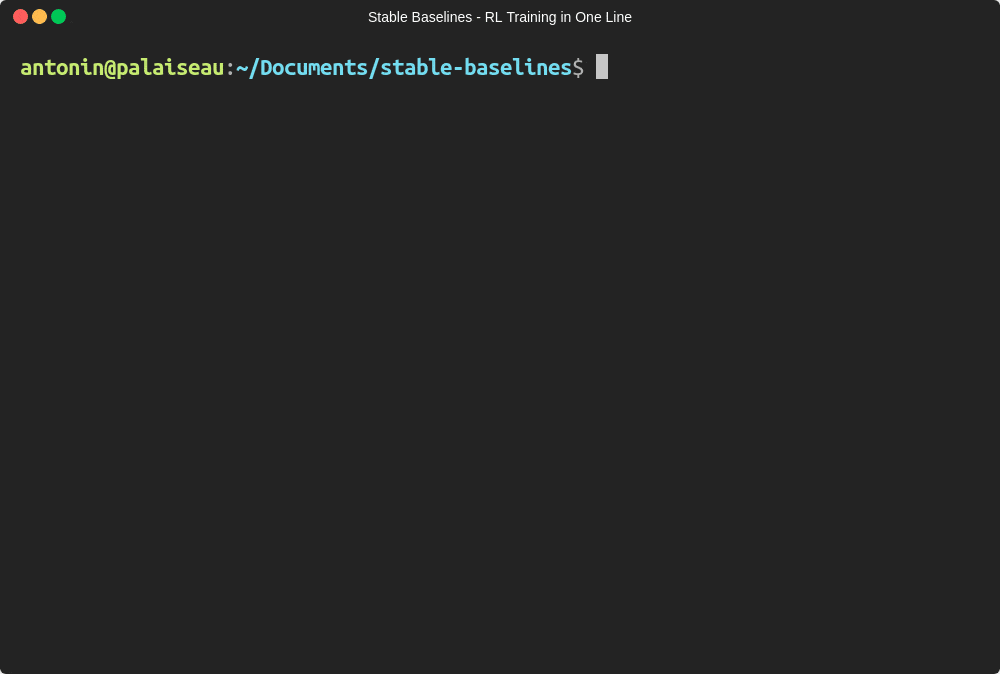# Getting Started¶

Most of the library tries to follow a sklearn-like syntax for the Reinforcement Learning algorithms.

Here is a quick example of how to train and run PPO2 on a cartpole environment:

import gym

from stable_baselines.common.policies import MlpPolicy
from stable_baselines.common.vec_env import DummyVecEnv
from stable_baselines import PPO2

env = gym.make('CartPole-v1')
# Optional: PPO2 requires a vectorized environment to run
# the env is now wrapped automatically when passing it to the constructor
# env = DummyVecEnv([lambda: env])

model = PPO2(MlpPolicy, env, verbose=1)
model.learn(total_timesteps=10000)

obs = env.reset()
for i in range(1000):
action, _states = model.predict(obs)
obs, rewards, dones, info = env.step(action)
env.render()


Or just train a model with a one liner if the environment is registered in Gym and if the policy is registered:

from stable_baselines import PPO2

model = PPO2('MlpPolicy', 'CartPole-v1').learn(10000)Define and train a RL agent in one line of code!